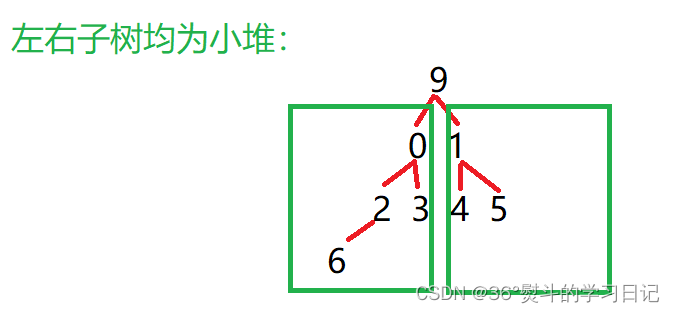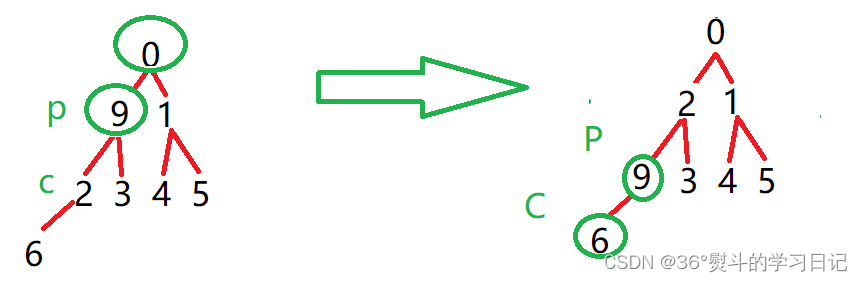﻿ C++超详细分析优化排序算法之堆排序_C 语言_脚本之家
C 语言# C++超详细分析优化排序算法之堆排序

1、向下调整算法

2、堆的逻辑结构是一棵完全二叉树

（以建小堆为例）看一种典型的示例：9 为父（根）节点，0，1都是其子节点，0 < 1；所以将0与9作一次交换；父节点同时下移至子节点，子节点变为新父节点的子节点：（p = parent, c = child）9 为父（根）节点，2，3都是其子节点，2 < 3；所以将2与9作一次交换；父节点同时下移至子节点，子节点变为新父节点的子节点：9 为父（根）节点，6 是其子节点，6< 9；所以将6与9作一次交换；父节点同时下移至子节点，子节点变为新父节点的子节点：leftchild = parent * 2 + 1;

rightchild = parent * 2 + 2;

parent = (leftchild - 1) /2;

```//向下调整：
//根节点左右子树必须已经成堆
void AdjustDown(int a[], int n, int parent)
{
int child = parent * 2 + 1;
//左孩子不能越界
while (child < n)
{
//如果只有左孩子，那就不用判断两个孩子的大小，直接判断左孩子和父亲的大小
if (child + 1 < n && a[child + 1] > a[child])
{
child++;
}
//向下调整
if (a[child] > a[parent])
{
Swap(&a[child], &a[parent]);
parent = child;
child = parent * 2 + 1;
}
else
{
break;
}
}
}```

```void Swap(int* a, int* b)
{
int tmp = *a;
*a = *b;
*b = tmp;
}``````//数组
int a[] = { 5,9,6,1,7,2,0,4,3,8 };
//元素个数
int n = (int)sizeof(a) / sizeof(a);```

```void HeapSort(int a[], int n)
{
//建堆
int parent = (n - 1 - 1) / 2;
while (parent >= 0)
{
parent--;
}
}```

```void HeapSort(int a[], int n)
{
//建堆
int parent = (n - 1 - 1) / 2;
while (parent >= 0)
{
parent--;
}
//已经成最大堆,不用再从最后一个父节点建堆
//每次只用改变根节点的堆（根左右堆已为最大堆）
int end = n - 1;
while (end > 0)
{
Swap(&a, &a[end]);
end--;
}
}```

```#include <stdio.h>
void Swap(int* a, int* b)
{
int tmp = *a;
*a = *b;
*b = tmp;
}
//向下调整：
//根节点左右子树必须已经成堆
void AdjustDown(int a[], int n, int parent)
{
int child = parent * 2 + 1;
//左孩子不能越界
while (child < n)
{
//如果只有左孩子，那就不用判断两个孩子的大小，直接判断左孩子和父亲的大小
if (child + 1 < n && a[child + 1] > a[child])
{
child++;
}
//向下调整
if (a[child] > a[parent])
{
Swap(&a[child], &a[parent]);
parent = child;
child = parent * 2 + 1;
}
else
{
break;
}
}
}
void HeapSort(int a[], int n)
{
int parent = (n - 1 - 1) / 2;
while (parent >= 0)
{
parent--;
}
//已经成最大堆,不用再从最后一个父节点建堆
//每次只用改变根节点的堆（根左右堆已为最大堆）
int end = n - 1;
while (end > 0)
{
Swap(&a, &a[end]);
end--;
}
}
void print(int* a, int n)
{
int i = 0;
for (i = 0; i < n; i++)
{
printf("%d ", a[i]);
}
printf("\n");
}
int main()
{
int a[] = { 5,9,6,1,7,2,0,4,3,8 };
int n = (int)sizeof(a) / sizeof(a);
HeapSort(a, n);
print(a, n);
return 0;
}```